### We have a double linked list where each node consists of three pointers data, random and next. Now our task is to clone this linked list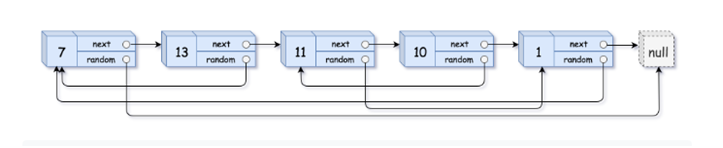Let’s first dive deep into the 1st solution of this problem

This method stores the next and random pointer (of original list) in an array first, then modifies the original Linked List (to create copy), creates a copy. And finally restores the original list.

1. First we will make a simple copy of the above linked list in which next is pointing to the next node and random is currently pointing to null.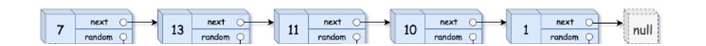2.   The next pointer of the original linked list will point to the node of the copied linked list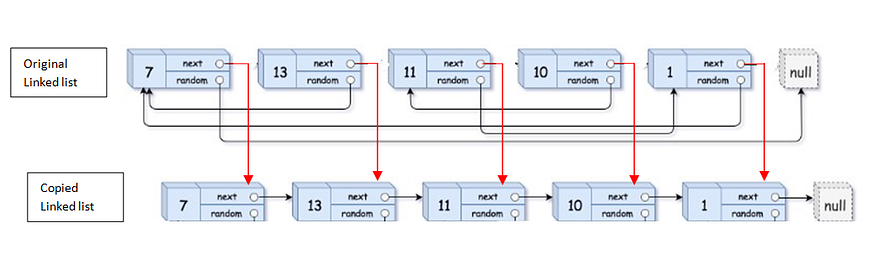3. For all random pointers in the copied linked list give address of respective nodes in the original linked list.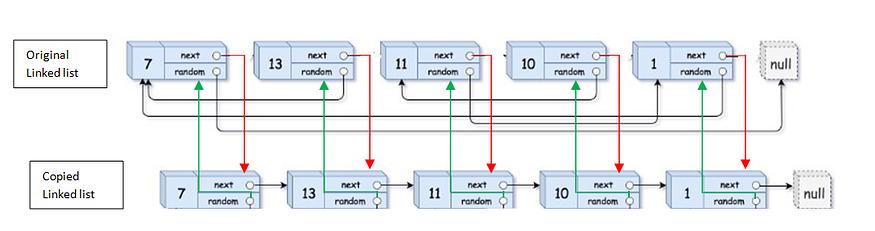4. Next we will perform the following statement

copied->random=copied->random->random->next

This will link the random pointers.

Now we will see how it works

copied->random

So let’s first see the left hand side of this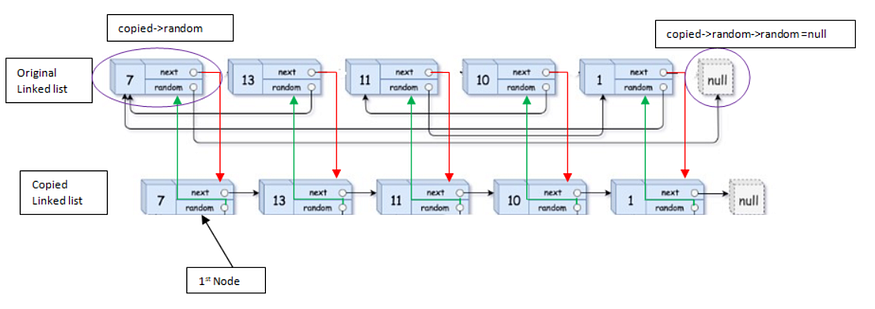So for 1st node

copied->random=null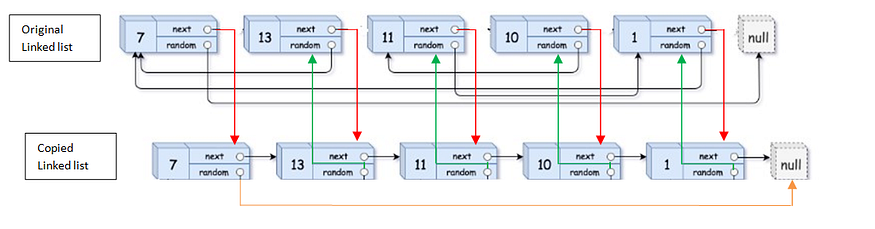let’s now look for the 2nd node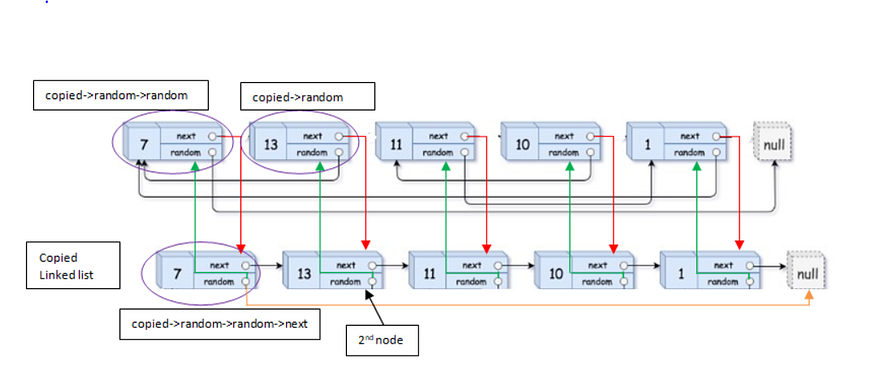From the above diagram it’s clear that the random pointer of 2nd node of copied linked list points to the first node which was actually same as in original linked list

So copied->random->random->next consists of 1st node

So RHS for 2nd node copied->random=address of the 1st node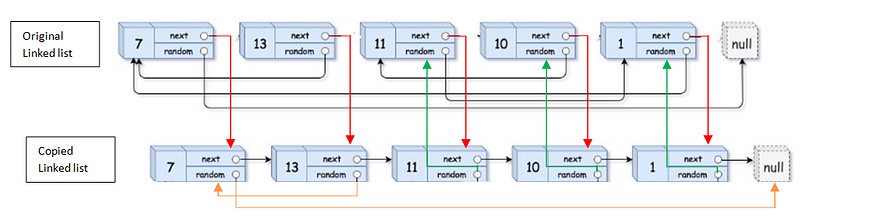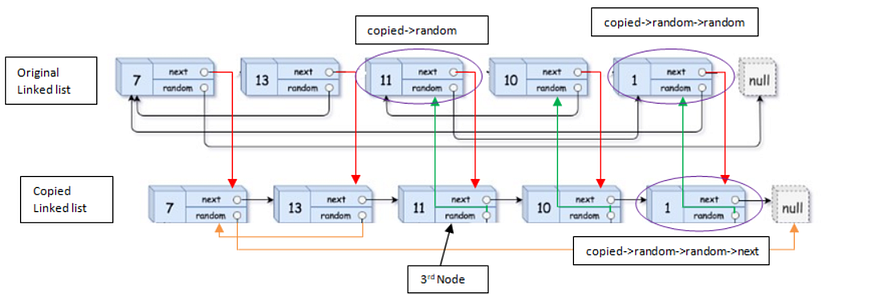So from the above diagram it is clear that random pointer 3rd Node of the copied list stores the 5th node of the copied list which is the same case as that of   the original one.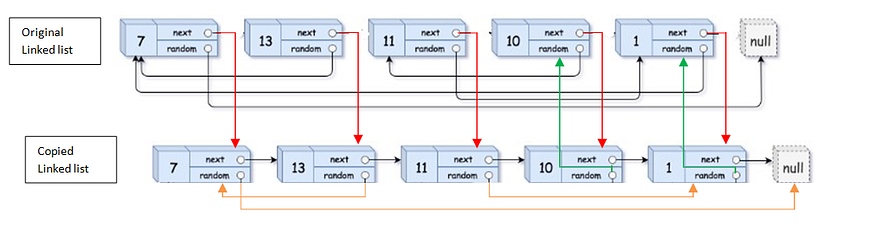Similarly it can be performed for rest of the nodes.

5. Restore the next pointer of the original linked list.

In this way we can clone a linked list consisting of a random pointer.

This will be the required cloned linked list.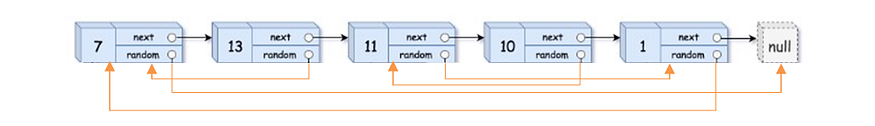## Complexity

Time Complexity: O (n)

Traversing once through the whole linked list.

Space Complexity: O (n)

Initially making a copy of the linked list of size n will occupy space with space complexity of O(n)

In my next blog I will take you through the another simple solution of this problem which has constant space complexity O (1)